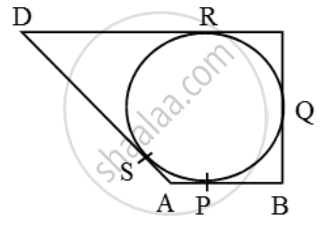Share

# A quadrilateral ABCD is drawn to circumscribe a circle, as shown in the figure. Prove that AB + CD = AD + BC - CBSE Class 10 - Mathematics

ConceptCircles Examples and Solutions

#### Question

A quadrilateral ABCD is drawn to circumscribe a circle, as shown in the figure. Prove that AB + CD = AD + BC

#### Solution

We known that the lengths of tangents drawn from an exterior point to a circle are equal.∴ AP = AS ….(i) [tangents from A]

BP = BQ ….(ii) [tangents from B]

CR = CQ ….(iii) [tangents from C]

DR = DS …(iv) [tangents from D]

∴ AB + CD = (AP + BP) + (CR + DR)

= (AS + BQ) + (CQ + DS) [using (i), (ii), (iii), (iv)]

= (AS + DS) + (BQ + CQ)